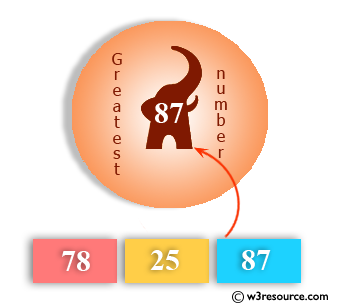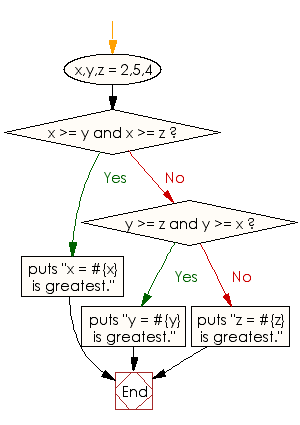﻿ Ruby Basic exercises: Find the greatest of three numbers - w3resource# Ruby Basic Exercises: Find the greatest of three numbers

## Ruby Basic: Exercise-20 with Solution

Write a Ruby program to find the greatest of three numbers.Ruby Code:

``````x,y,z = 2,5,4
if x >= y and x >= z
puts "x = #{x} is greatest."
elsif y >= z and y >= x
puts "y = #{y} is greatest."
else
puts "z = #{z} is greatest."
end
``````

Output:

```y = 5 is greatest.
```

Flowchart:Ruby Code Editor: